CGAL 5.1 - 2D Triangulation
CGAL::Triangulation_2< Traits, Tds > Class Template Reference

#include <CGAL/Triangulation_2.h>

## Definition

The class Triangulation_2 is the basic class designed to handle triangulations of set of points $${ A}$$ in the plane.

Such a triangulation has vertices at the points of $${ A}$$ and its domain covers the convex hull of $${ A}$$. It can be viewed as a planar partition of the plane whose bounded faces are triangular and cover the convex hull of $${ A}$$. The single unbounded face of this partition is the complementary of the convex hull of $${ A}$$.

In many applications, it is convenient to deal only with triangular faces. Therefore, we add to the triangulation a fictitious vertex, called the infinite vertex and we make each convex hull edge incident to an infinite face having as third vertex the infinite vertex. In that way, each edge is incident to exactly two faces and special cases at the boundary of the convex hull are simpler to deal with.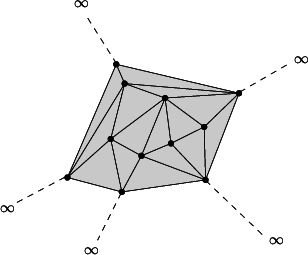The infinite vertex.
The class Triangulation_2 implements this point of view and therefore considers the triangulation of the set of points as a set of triangular, finite and infinite faces. Although it is convenient to draw a triangulation as in figure Triangulation_ref_Fig_infinite_vertex, note that the infinite vertex has no significant coordinates and that no geometric predicate can be applied on it or on an infinite face.

A triangulation is a collection of vertices and faces that are linked together through incidence and adjacency relations. Each face give access to its three incident vertices and to its three adjacent faces. Each vertex give access to one of its incident faces.

The three vertices of a face are indexed with 0, 1 and 2 in counterclockwise order. The neighbor of a face are also indexed with 0,1,2 in such a way that the neighbor indexed by $$i$$ is opposite to the vertex with the same index.

The triangulation class offers two functions int cw(int i) and int ccw(int i) which, given the index of a vertex in a face, compute the index of the next vertex of the same face in clockwise or counterclockwise order. Thus, for example the neighbor neighbor(cw(i)) is the neighbor of f which is next to neighbor(i) turning clockwise around f. The face neighbor(cw(i)) is also the first face encountered after f when turning clockwise around vertex i of f (see Figure Triangulation_ref_Fig_neighbors).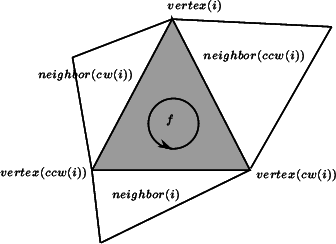Vertices and neighbors.
Template Parameters
 Traits is the geometric traits which must be a model of the concept TriangulationTraits_2. Tds is the triangulation data structure which must be a model of the concept TriangulationDataStructure_2. By default, the triangulation data structure is instantiated by Triangulation_data_structure_2 < Triangulation_vertex_base_2, Triangulation_face_base_2 >.

Traversal of the Triangulation

A triangulation can be seen as a container of faces and vertices. Therefore the triangulation provides several iterators and circulators that allow to traverse it completely or partially.

Traversal of the Convex Hull

Applied on the infinite vertex the above functions allow to visit the vertices on the convex hull and the infinite edges and faces. Note that a counterclockwise traversal of the vertices adjacent to the infinite vertex is a clockwise traversal of the convex hull.

Face_circulator incident_faces(t.infinite_vertex()) const;
Face_circulator incident_faces(t.infinite_vertex(), f) const;
Edge_circulator incident_edges(t.infinite_vertex()) const;
Edge_circulator incident_edges(t.infinite_vertex(), f);
Vertex_circulator incident_vertices(t.infinite_vertex() v) ;
Vertex_circulator incident_vertices(t.infinite_vertex(), f) ;

I/O

The I/O operators are defined for iostream. The format for the iostream is an internal format.

The information output in the iostream is:

• the number of vertices (including the infinite one), the number of faces (including infinite ones), and the dimension.
• for each vertex (except the infinite vertex), the non combinatorial information stored in that vertex (point, etc.).
• for each faces, the indices of its vertices and the non combinatorial information (if any) in this face.
• for each face again the indices of the neighboring faces.

The index of an item (vertex of face) is the rank of this item in the output order. When dimension $$<$$ 2, the same information is output for faces of maximal dimension instead of faces.

Implementation

Locate is implemented by a line walk from a vertex of the face given as optional parameter (or from a finite vertex of infinite_face() if no optional parameter is given). It takes time $$O(n)$$ in the worst case, but only $$O(\sqrt{n})$$ on average if the vertices are distributed uniformly at random.

Insertion of a point is done by locating a face that contains the point, and then splitting this face. If the point falls outside the convex hull, the triangulation is restored by flips. Apart from the location, insertion takes a time time $$O(1)$$. This bound is only an amortized bound for points located outside the convex hull.

Removal of a vertex is done by removing all adjacent triangles, and re-triangulating the hole. Removal takes time $$O(d^2)$$ in the worst case, if $$d$$ is the degree of the removed vertex, which is $$O(1)$$ for a random vertex.

The face, edge, and vertex iterators on finite features are derived from their counterparts visiting all (finite and infinite) features which are themselves derived from the corresponding iterators of the triangulation data structure.

TriangulationTraits_2
TriangulationDataStructure_2
TriangulationDataStructure_2::Face
TriangulationDataStructure_2::Vertex
CGAL::Triangulation_data_structure_2<Vb,Fb>
CGAL::Triangulation_vertex_base_2<Traits>
CGAL::Triangulation_face_base_2<Traits>
Examples:
Triangulation_2/adding_handles.cpp, Triangulation_2/colored_face.cpp, Triangulation_2/draw_triangulation_2.cpp, Triangulation_2/for_loop_2.cpp, Triangulation_2/low_dimensional.cpp, and Triangulation_2/triangulation_prog1.cpp.

## Related Functions

(Note that these are not member functions.)

ostream & operator<< (ostream &os, const Triangulation_2< Traits, Tds > &T)
Inserts the triangulation into the stream os. More...

istream & operator>> (istream &is, const Triangulation_2< Traits, Tds > &T)
Reads a triangulation from stream is and assigns it to the triangulation. More...

## Types

typedef Traits Geom_traits
the traits class.

typedef Tds Triangulation_data_structure
the triangulation data structure type.

typedef Traits::Point_2 Point
the point type.

typedef Traits::Segment_2 Segment
the segment type.

typedef Traits::Triangle_2 Triangle
the triangle type.

typedef Tds::Vertex Vertex
the vertex type.

typedef Tds::Face Face
the face type.

typedef Tds::Edge Edge
the edge type.

typedef Tds::size_type size_type
Size type (an unsigned integral type).

typedef Tds::difference_type difference_type
Difference type (a signed integral type).

## Handles, Iterators, and Circulators

The vertices and faces of the triangulations are accessed through handles, iterators and circulators.

The handles are models of the concept Handle which basically offers the two dereference operators and ->. The handles are also model of the concepts LessThanComparable and Hashable, that is they can be used as keys in containers such as std::map and boost::unordered_map. The iterators and circulators are all bidirectional and non-mutable. The circulators and iterators are convertible to handles with the same value type, so that whenever a handle appear in the parameter list of a function, an appropriate iterator or circulator can be passed as well.

The edges of the triangulation can also be visited through iterators and circulators, the edge circulators and iterators are also bidirectional and non mutable.

In the following, we called infinite any face or edge incident to the infinite vertex and the infinite vertex itself. Any other feature (face, edge or vertex) of the triangulation is said to be finite. Some iterators (the All iterators ) allows to visit finite or infinite feature while others (the Finite iterators) visit only finite features. Circulators visit infinite features as well as finite ones. The triangulation class also defines the following enum type to specify which case occurs when locating a point in the triangulation.

In order to write C++ 11 for-loops we provide range types.

enum  Locate_type {
VERTEX =0, EDGE, FACE, OUTSIDE_CONVEX_HULL,
OUTSIDE_AFFINE_HULL
}
specifies which case occurs when locating a point in the triangulation. More...

typedef Tds::Vertex_handle Vertex_handle
handle to a vertex.

typedef Tds::Face_handle Face_handle
handle to a face.

typedef Tds::Face_iterator All_faces_iterator
iterator over all faces.

typedef Tds::Edge_iterator All_edges_iterator
iterator over all edges.

typedef Tds::Vertex_iterator All_vertices_iterator
iterator over all vertices.

typedef unspecified_type Finite_faces_iterator
iterator over finite faces.

typedef unspecified_type Finite_edges_iterator
iterator over finite edges.

typedef unspecified_type Finite_vertices_iterator
iterator over finite vertices.

typedef unspecified_type Point_iterator
iterator over the points corresponding the finite vertices of the triangulation.

typedef Iterator_range< unspecified_typeAll_face_handles
range type for iterating over all faces (including infinite faces), with a nested type iterator that has as value type Face_handle

typedef Iterator_range< All_edges_iteratorAll_edges
range type for iterating over all edges (including infinite ones).

typedef Iterator_range< unspecified_typeAll_vertex_handles
range type for iterating over all vertices (including the infinite vertex), with a nested type iterator that has as value type Vertex_handle

typedef Iterator_range< unspecified_typeFinite_face_handles
range type for iterating over finite faces, with a nested type iterator that has as value type Face_handle

typedef Iterator_range< Finite_edges_iteratorFinite_edges
range type for iterating over finite edges.

typedef Iterator_range< unspecified_typeFinite_vertex_handles
range type for iterating over finite vertices, with a nested type iterator that has as value type Vertex_handle

typedef Iterator_range< Point_iteratorPoints
range type for iterating over the points of the finite vertices.

typedef unspecified_type Line_face_circulator
circulator over all faces intersected by a line.

typedef unspecified_type Face_circulator
circulator over all faces incident to a given vertex.

typedef unspecified_type Edge_circulator
circulator over all edges incident to a given vertex.

typedef unspecified_type Vertex_circulator
circulator over all vertices incident to a given vertex.

## Creation

Triangulation_2 (const Traits &gt=Traits())
Introduces an empty triangulation.

Triangulation_2 (const Triangulation_2 &tr)
Copy constructor. More...

template<class InputIterator >
Triangulation_2 (InputIterator first, InputIterator last, const Traits &gt=Traits())
Equivalent to constructing an empty triangulation with the optional traits class argument and calling insert(first,last).

Triangulation_2 operator= (const Triangulation_2< Traits, Tds > &tr)
Assignment. More...

void swap (Triangulation_2 &tr)
The triangulations tr and *this are swapped. More...

void clear ()
Deletes all faces and finite vertices resulting in an empty triangulation.

## Access Functions

int dimension () const
Returns the dimension of the convex hull.

size_type number_of_vertices () const
Returns the number of finite vertices.

size_type number_of_faces () const
Returns the number of finite faces.

Face_handle infinite_face () const
a face incident to the infinite vertex.

Vertex_handle infinite_vertex () const
the infinite vertex.

Vertex_handle finite_vertex () const
a vertex distinct from the infinite vertex.

const Geom_traitsgeom_traits () const
Returns a const reference to the triangulation traits object.

const TriangulationDataStructure_2tds () const
Returns a const reference to the triangulation data structure.

## Non const access

Attention
The responsibility of keeping a valid triangulation belongs to the user when using advanced operations allowing a direct manipulation of the tds. This method is mainly a help for users implementing their own triangulation algorithms.
TriangulationDataStructure_2tds ()
Returns a reference to the triangulation data structure.

## Predicates

The class Triangulation_2 provides methods to test the finite or infinite character of any feature, and also methods to test the presence in the triangulation of a particular feature (edge or face).

bool is_infinite (Vertex_handle v) const
true iff v is the infinite vertex.

bool is_infinite (Face_handle f) const
true iff face f is infinite.

bool is_infinite (Face_handle f, int i) const
true iff edge (f,i) is infinite.

bool is_infinite (Edge e) const
true iff edge e is infinite.

bool is_infinite (Edge_circulator ec) const
true iff edge *ec is infinite.

bool is_infinite (All_edges_iterator ei) const
true iff edge *ei is infinite.

bool is_edge (Vertex_handle va, Vertex_handle vb)
true if there is an edge having va and vb as vertices.

bool is_edge (Vertex_handle va, Vertex_handle vb, Face_handle &fr, int &i)
as above. More...

bool includes_edge (Vertex_handle va, Vertex_handle vb, Vertex_handle &vbr, Face_handle &fr, int &i)
true if the line segment from va to vb includes an edge e incident to va. More...

bool is_face (Vertex_handle v1, Vertex_handle v2, Vertex_handle v3)
true if there is a face having v1, v2 and v3 as vertices.

bool is_face (Vertex_handle v1, Vertex_handle v2, Vertex_handle v3, Face_handle &fr)
as above. More...

## Queries

The class Triangulation_2 provides methods to locate a given point with respect to a triangulation.

It also provides methods to locate a point with respect to a given finite face of the triangulation.

Face_handle locate (const Point &query, Face_handle f=Face_handle()) const
If the point query lies inside the convex hull of the points, a face that contains the query in its interior or on its boundary is returned. More...

Face_handle inexact_locate (const Point &query, Face_handle start=Face_handle()) const
Same as locate() but uses inexact predicates. More...

Face_handle locate (const Point &query, Locate_type &lt, int &li, Face_handle h=Face_handle()) const
Same as above. More...

Oriented_side oriented_side (Face_handle f, const Point &p) const
Returns on which side of the oriented boundary of f lies the point p. More...

Oriented_side side_of_oriented_circle (Face_handle f, const Point &p)
Returns on which side of the circumcircle of face f lies the point p. More...

## Modifiers

The following operations are guaranteed to lead to a valid triangulation when they are applied on a valid triangulation.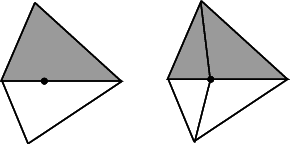Insertion of a point on an edge.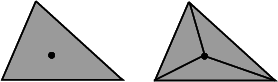Insertion in a face.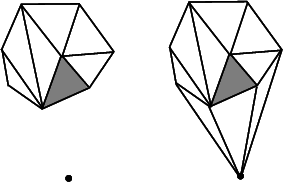Insertion outside the convex hull.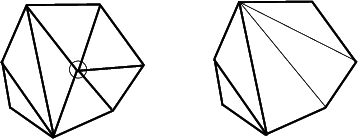Removal.
void flip (Face_handle f, int i)
Exchanges the edge incident to f and f->neighbor(i) with the other diagonal of the quadrilateral formed by f and f->neighbor(i). More...

Vertex_handle insert (const Point &p, Face_handle f=Face_handle())
Inserts point p in the triangulation and returns the corresponding vertex. More...

Vertex_handle insert (const Point &p, Locate_type lt, Face_handle loc, int li)
Same as above except that the location of the point p to be inserted is assumed to be given by (lt,loc,i) (see the description of the locate method above.)

Vertex_handle push_back (const Point &p)
Equivalent to insert(p).

template<class PointInputIterator >
std::ptrdiff_t insert (PointInputIterator first, PointInputIterator last)
Inserts the points in the range [first,last) in the given order, and returns the number of inserted points. More...

template<class PointWithInfoInputIterator >
std::ptrdiff_t insert (PointWithInfoInputIterator first, PointWithInfoInputIterator last)
inserts the points in the iterator range [first,last) in the given order, and returns the number of inserted points. More...

void remove (Vertex_handle v)
Removes the vertex from the triangulation. More...

Vertex_handle move_if_no_collision (Vertex_handle v, const Point &p)
If there is not already another vertex placed on p, the triangulation is modified such that the new position of vertex v is p, and v is returned. More...

Vertex_handle move (Vertex_handle v, const Point &p)
If there is no collision during the move, this function is the same as move_if_no_collision . More...

## Specialized Modifiers

The following member functions offer more specialized versions of the insertion or removal operations to be used when one knows to be in the corresponding case.

Vertex_handle insert_first (const Point &p)
Inserts the first finite vertex .

Vertex_handle insert_second (const Point &p)
Inserts the second finite vertex .

Vertex_handle insert_in_face (const Point &p, Face_handle f)
Inserts vertex v in face f. More...

Vertex_handle insert_in_edge (const Point &p, Face_handle f, int i)
Inserts vertex v in edge i of f. More...

Vertex_handle insert_outside_convex_hull (const Point &p, Face_handle f)
Inserts a point which is outside the convex hull but in the affine hull. More...

Vertex_handle insert_outside_affine_hull (const Point &p)
Inserts a point which is outside the affine hull.

void remove_degree_3 (Vertex_handle v)
Removes a vertex of degree three. More...

void remove_second (Vertex_handle v)
Removes the before last finite vertex.

void remove_first (Vertex_handle v)
Removes the last finite vertex.

template<class EdgeIt >
Vertex_handle star_hole (Point p, EdgeIt edge_begin, EdgeIt edge_end)
creates a new vertex v and use it to star the hole whose boundary is described by the sequence of edges [edge_begin, edge_end). More...

template<class EdgeIt , class FaceIt >
Vertex_handle star_hole (Point p, EdgeIt edge_begin, EdgeIt edge_end, FaceIt face_begin, FaceIt face_end)
same as above, except that the algorithm first recycles faces in the sequence [face_begin, face_end) and create new ones only when the sequence is exhausted. More...

## Finite Face, Edge and Vertex Iterators

The following iterators allow respectively to visit finite faces, finite edges and finite vertices of the triangulation.

These iterators are non mutable, bidirectional and their value types are respectively Face, Edge and Vertex. They are all invalidated by any change in the triangulation.

Finite_vertices_iterator finite_vertices_begin () const
Starts at an arbitrary finite vertex.

Finite_vertices_iterator finite_vertices_end () const
Past-the-end iterator.

Finite_edges_iterator finite_edges_begin () const
Starts at an arbitrary finite edge.

Finite_edges_iterator finite_edges_end () const
Past-the-end iterator.

Finite_faces_iterator finite_faces_begin () const
Starts at an arbitrary finite face.

Finite_faces_iterator finite_faces_end () const
Past-the-end iterator.

Point_iterator points_begin () const

Point_iterator points_end () const
Past-the-end iterator.

Finite_vertex_handles finite_vertex_handles () const
returns a range of iterators over finite vertices. More...

Finite_edges finite_edges () const
returns a range of iterators over finite edges.

Finite_face_handles finite_face_handles () const
returns a range of iterators over finite faces. More...

Points points () const
returns a range of iterators over the points of finite vertices.

## All Face, Edge and Vertex Iterators

The following iterators allow respectively to visit all (finite or infinite) faces, edges and vertices of the triangulation.

These iterators are non mutable, bidirectional and their value types are respectively Face, Edge and Vertex. They are all invalidated by any change in the triangulation.

All_vertices_iterator all_vertices_begin () const
Starts at an arbitrary vertex.

All_vertices_iterator all_vertices_end () const
Past-the-end iterator.

All_edges_iterator all_edges_begin () const
Starts at an arbitrary edge.

All_edges_iterator all_edges_end () const
Past-the-end iterator.

All_faces_iterator all_faces_begin () const
Starts at an arbitrary face.

All_faces_iterator all_faces_end () const
Past-the-end iterator.

All_vertex_handles all_vertex_handles () const
returns a range of iterators over all vertices. More...

All_edges all_edges () const
returns a range of iterators over all edges.

All_face_handles all_face_handles () const
returns a range of iterators over all faces. More...

## Line Face Circulator

The triangulation defines a circulator that allows to visit all faces that are intersected by a line.

A face f is considered has being intersected by the oriented line l if either:

• f is a finite face whose interior intersects l, or
• f is a finite face with an edge collinear with l and lies to the left of l, or
• f is an infinite face incident to a convex hull edge whose interior is intersected by l, or
• f is an infinite face incident to a convex hull vertex lying on l and the finite edge of f lies to the left of l.

The circulator has a singular value if the line l intersect no finite face of the triangulation. This circulator is non-mutable and bidirectional. Its value type is Face. Figure Triangulation_ref_Fig_Line_face_circulator illustrates which finite faces are enumerated. Lines $$l_1$$ and $$l_2$$ have no face to their left. Lines $$l_3$$ and $$l_4$$ have faces to their left. Note that the finite faces that are only vertex incident to lines $$l_3$$ and $$l_4$$ are not enumerated.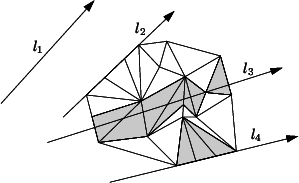The line face circulator. A line face circulator is invalidated if the face the circulator refers to is changed.
Line_face_circulator line_walk (const Point &p, const Point &q, Face_handle f=Face_handle()) const
This function returns a circulator that allows to visit the faces intersected by the line pq. More...

## Face, Edge and Vertex Circulators

The triangulation also provides circulators that allows to visit respectively all faces or edges incident to a given vertex or all vertices adjacent to a given vertex.

These circulators are non-mutable and bidirectional. The operator++ moves the circulator counterclockwise around the vertex while the operator- moves clockwise. A face circulator is invalidated by any modification of the face pointed to. An edge or a vertex circulator are invalidated by any modification of one of the two faces incident to the edge pointed to.

Face_circulator incident_faces (Vertex_handle v) const
Starts at an arbitrary face incident to v.

Face_circulator incident_faces (Vertex_handle v, Face_handle f) const
Starts at face f. More...

Edge_circulator incident_edges (Vertex_handle v) const
Starts at an arbitrary edge incident to v.

Edge_circulator incident_edges (Vertex_handle v, Face_handle f) const
Starts at the first edge of f incident to v, in counterclockwise order around v. More...

Vertex_circulator incident_vertices (Vertex_handle v) const
Starts at an arbitrary vertex incident to v.

Vertex_circulator incident_vertices (Vertex_handle v, Face_handle f)
Starts at the first vertex of f adjacent to v in counterclockwise order around v. More...

Vertex_handle mirror_vertex (Face_handle f, int i) const
returns the vertex of the $$i^{th}$$ neighbor of f that is opposite to f. More...

int mirror_index (Face_handle f, int i) const
returns the index of f in its $$i^{th}$$ neighbor. More...

Edge mirror_edge (Edge e) const
returns the same edge seen from the other adjacent face. More...

## Miscellaneous

int ccw (int i) const
Returns $$i+1$$ modulo 3. More...

int cw (int i) const
Returns $$i+2$$ modulo 3. More...

Triangle triangle (Face_handle f) const
Returns the triangle formed by the three vertices of f. More...

Segment segment (Face_handle f, int i) const
Returns the line segment formed by the vertices ccw(i) and cw(i) of face f. More...

Segment segment (const Edge &e) const
Returns the line segment corresponding to edge e. More...

Segment segment (const Edge_circulator &ec) const
Returns the line segment corresponding to edge *ec. More...

Segment segment (const Edge_iterator &ei) const
Returns the line segment corresponding to edge *ei. More...

Point circumcenter (Face_handle f) const
Compute the circumcenter of the face pointed to by f. More...

## Setting

void set_infinite_vertex (const Vertex_handle &v)
This is an advanced function. More...

## Checking

The responsibility of keeping a valid triangulation belongs to the users if advanced operations are used.

Obviously the advanced user, who implements higher levels operations may have to make a triangulation invalid at some times. The following method is provided to help the debugging.

bool is_valid (bool verbose=false, int level=0) const
Checks the combinatorial validity of the triangulation and also the validity of its geometric embedding. More...Public Member Functions inherited from CGAL::Triangulation_cw_ccw_2
Triangulation_cw_ccw_2 ()
default constructor.

int ccw (const int i) const
returns the index of the neighbor or vertex that is next to the neighbor or vertex with index i in counterclockwise order around a face.

int cw (const int i) const
returns the index of the neighbor or vertex that is next to the neighbor or vertex with index i in counterclockwise order around a face.

## ◆ Locate_type

template<typename Traits , typename Tds >

specifies which case occurs when locating a point in the triangulation.

CGAL::Triangulation_2<Traits,Tds>
Enumerator
VERTEX

when the located point coincides with a vertex of the triangulation

EDGE

when the point is in the relative interior of an edge

FACE

when the point is in the interior of a facet

OUTSIDE_CONVEX_HULL

when the point is outside the convex hull but in the affine hull of the current triangulation

OUTSIDE_AFFINE_HULL

when the point is outside the affine hull of the current triangulation.

## ◆ Triangulation_2()

template<typename Traits , typename Tds >
 CGAL::Triangulation_2< Traits, Tds >::Triangulation_2 ( const Triangulation_2< Traits, Tds > & tr )

Copy constructor.

All the vertices and faces are duplicated. After the copy, *this and tr refer to different triangulations: if tr is modified, *this is not.

## ◆ all_face_handles()

template<typename Traits , typename Tds >
 All_face_handles CGAL::Triangulation_2< Traits, Tds >::all_face_handles ( ) const

returns a range of iterators over all faces.

Note
While the value type of All_faces_iterator is Face, the value type of All_face_handles::iterator is Face_handle

## ◆ all_vertex_handles()

template<typename Traits , typename Tds >
 All_vertex_handles CGAL::Triangulation_2< Traits, Tds >::all_vertex_handles ( ) const

returns a range of iterators over all vertices.

Note
While the value type of All_vertices_iterator is Vertex, the value type of All_vertex_handles::iterator is Vertex_handle

## ◆ ccw()

template<typename Traits , typename Tds >
 int CGAL::Triangulation_2< Traits, Tds >::ccw ( int i ) const

Returns $$i+1$$ modulo 3.

Precondition
$$0\leq i \leq2$$.

## ◆ circumcenter()

template<typename Traits , typename Tds >
 Point CGAL::Triangulation_2< Traits, Tds >::circumcenter ( Face_handle f ) const

Compute the circumcenter of the face pointed to by f.

This function is available only if the corresponding function is provided in the geometric traits.

## ◆ cw()

template<typename Traits , typename Tds >
 int CGAL::Triangulation_2< Traits, Tds >::cw ( int i ) const

Returns $$i+2$$ modulo 3.

Precondition
$$0\leq i \leq2$$.

## ◆ finite_face_handles()

template<typename Traits , typename Tds >
 Finite_face_handles CGAL::Triangulation_2< Traits, Tds >::finite_face_handles ( ) const

returns a range of iterators over finite faces.

Note
While the value type of Finite_faces_iterator is Face, the value type of Finite_face_handles::iterator is Face_handle

## ◆ finite_vertex_handles()

template<typename Traits , typename Tds >
 Finite_vertex_handles CGAL::Triangulation_2< Traits, Tds >::finite_vertex_handles ( ) const

returns a range of iterators over finite vertices.

Note
While the value type of Finite_vertices_iterator is Vertex, the value type of Finite_vertex_handles::iterator is Vertex_handle

## ◆ flip()

template<typename Traits , typename Tds >
 void CGAL::Triangulation_2< Traits, Tds >::flip ( Face_handle f, int i )

Exchanges the edge incident to f and f->neighbor(i) with the other diagonal of the quadrilateral formed by f and f->neighbor(i).

Precondition
The faces f and f->neighbor(i) are finite faces and their union form a convex quadrilateral.

## ◆ incident_edges()

template<typename Traits , typename Tds >
 Edge_circulator CGAL::Triangulation_2< Traits, Tds >::incident_edges ( Vertex_handle v, Face_handle f ) const

Starts at the first edge of f incident to v, in counterclockwise order around v.

Precondition
Face f is incident to vertex v.

## ◆ incident_faces()

template<typename Traits , typename Tds >
 Face_circulator CGAL::Triangulation_2< Traits, Tds >::incident_faces ( Vertex_handle v, Face_handle f ) const

Starts at face f.

Precondition
Face f is incident to vertex v.

## ◆ incident_vertices()

template<typename Traits , typename Tds >
 Vertex_circulator CGAL::Triangulation_2< Traits, Tds >::incident_vertices ( Vertex_handle v, Face_handle f )

Starts at the first vertex of f adjacent to v in counterclockwise order around v.

Precondition
Face f is incident to vertex v.

## ◆ includes_edge()

template<typename Traits , typename Tds >
 bool CGAL::Triangulation_2< Traits, Tds >::includes_edge ( Vertex_handle va, Vertex_handle vb, Vertex_handle & vbr, Face_handle & fr, int & i )

true if the line segment from va to vb includes an edge e incident to va.

If true, vbr becomes the other vertex of e, e is the edge (fr,i) where fr is a handle to the face incident to e and on the right side e oriented from va to vb.

## ◆ inexact_locate()

template<typename Traits , typename Tds >
 Face_handle CGAL::Triangulation_2< Traits, Tds >::inexact_locate ( const Point & query, Face_handle start = Face_handle() ) const

Same as locate() but uses inexact predicates.

This function returns a handle on a face that is a good approximation of the exact location of query, while being faster. Note that it may return a handle on a face whose interior does not contain query. When the triangulation has dimension smaller than 2, start is returned.

## ◆ insert() [1/3]

template<typename Traits , typename Tds >
 Vertex_handle CGAL::Triangulation_2< Traits, Tds >::insert ( const Point & p, Face_handle f = Face_handle() )

Inserts point p in the triangulation and returns the corresponding vertex.

If point p coincides with an already existing vertex, this vertex is returned and the triangulation remains unchanged.

If point p is on an edge, the two incident faces are split in two.

If point p is strictly inside a face of the triangulation, the face is split in three.

If point p is strictly outside the convex hull, p is linked to all visible points on the convex hull to form the new triangulation.

At last, if p is outside the affine hull (in case of degenerate 1-dimensional or 0-dimensional triangulations), p is linked all the other vertices to form a triangulation whose dimension is increased by one. The last argument f is an indication to the underlying locate algorithm of where to start.

Examples:

## ◆ insert() [2/3]

template<typename Traits , typename Tds >
template<class PointInputIterator >
 std::ptrdiff_t CGAL::Triangulation_2< Traits, Tds >::insert ( PointInputIterator first, PointInputIterator last )

Inserts the points in the range [first,last) in the given order, and returns the number of inserted points.

Template Parameters
 PointInputIterator must be an input iterator with value type Point.

## ◆ insert() [3/3]

template<typename Traits , typename Tds >
template<class PointWithInfoInputIterator >
 std::ptrdiff_t CGAL::Triangulation_2< Traits, Tds >::insert ( PointWithInfoInputIterator first, PointWithInfoInputIterator last )

inserts the points in the iterator range [first,last) in the given order, and returns the number of inserted points.

Given a pair (p,i), the vertex v storing p also stores i, that is v.point() == p and v.info() == i. If several pairs have the same point, only one vertex is created, and one of the objects of type Vertex::Info will be stored in the vertex.

Precondition
Vertex must be model of the concept TriangulationVertexBaseWithInfo_2.
Template Parameters
 PointWithInfoInputIterator must be an input iterator with the value type std::pair.

## ◆ insert_in_edge()

template<typename Traits , typename Tds >
 Vertex_handle CGAL::Triangulation_2< Traits, Tds >::insert_in_edge ( const Point & p, Face_handle f, int i )

Inserts vertex v in edge i of f.

Precondition
The point in vertex v lies on the edge opposite to the vertex i of face f.

## ◆ insert_in_face()

template<typename Traits , typename Tds >
 Vertex_handle CGAL::Triangulation_2< Traits, Tds >::insert_in_face ( const Point & p, Face_handle f )

Inserts vertex v in face f.

Face f is modified, two new faces are created.

Precondition
The point in vertex v lies inside face f.

## ◆ insert_outside_convex_hull()

template<typename Traits , typename Tds >
 Vertex_handle CGAL::Triangulation_2< Traits, Tds >::insert_outside_convex_hull ( const Point & p, Face_handle f )

Inserts a point which is outside the convex hull but in the affine hull.

Precondition
The handle f points to a face which is a proof of the location ofp, see the description of the locate method above.

## ◆ is_edge()

template<typename Traits , typename Tds >
 bool CGAL::Triangulation_2< Traits, Tds >::is_edge ( Vertex_handle va, Vertex_handle vb, Face_handle & fr, int & i )

as above.

In addition, if true is returned, the edge with vertices va and vb is the edge e=(fr,i) where fr is a handle to the face incident to e and on the right side of e oriented from va to vb.

## ◆ is_face()

template<typename Traits , typename Tds >
 bool CGAL::Triangulation_2< Traits, Tds >::is_face ( Vertex_handle v1, Vertex_handle v2, Vertex_handle v3, Face_handle & fr )

as above.

In addition, if true is returned, fr is a handle to the face with v1, v2 and v3 as vertices.

## ◆ is_valid()

template<typename Traits , typename Tds >
 bool CGAL::Triangulation_2< Traits, Tds >::is_valid ( bool verbose = false, int level = 0 ) const

Checks the combinatorial validity of the triangulation and also the validity of its geometric embedding.

This method is mainly a debugging help for the users of advanced features.

## ◆ line_walk()

template<typename Traits , typename Tds >
 Line_face_circulator CGAL::Triangulation_2< Traits, Tds >::line_walk ( const Point & p, const Point & q, Face_handle f = Face_handle() ) const

This function returns a circulator that allows to visit the faces intersected by the line pq.

If there is no such face the circulator has a singular value.

The starting point of the circulator is the face f, or the first finite face traversed by l , if f is omitted.

The circulator wraps around the infinite vertex: after the last traversed finite face, it steps through the infinite face adjacent to this face then through the infinite face adjacent to the first traversed finite face then through the first finite traversed face again.

Precondition
The triangulation must have dimension 2.
Points p and q must be different points.
If f != nullptr, it must point to a finite face and the point p must be inside or on the boundary of f.

## ◆ locate() [1/2]

template<typename Traits , typename Tds >
 Face_handle CGAL::Triangulation_2< Traits, Tds >::locate ( const Point & query, Face_handle f = Face_handle() ) const

If the point query lies inside the convex hull of the points, a face that contains the query in its interior or on its boundary is returned.

If the point query lies outside the convex hull of the triangulation but in the affine hull, the returned face is an infinite face which is a proof of the point's location:

• for a two dimensional triangulation, it is a face $$(\infty, p, q)$$ such that query lies to the left of the oriented line $$pq$$ (the rest of the triangulation lying to the right of this line).
• for a degenerate one dimensional triangulation it is the (degenerate one dimensional) face $$(\infty, p, nullptr)$$ such that query and the triangulation lie on either side of p.

If the point query lies outside the affine hull, the returned Face_handle is nullptr.

The optional Face_handle argument, if provided, is used as a hint of where the locate process has to start its search.

## ◆ locate() [2/2]

template<typename Traits , typename Tds >
 Face_handle CGAL::Triangulation_2< Traits, Tds >::locate ( const Point & query, Locate_type & lt, int & li, Face_handle h = Face_handle() ) const

Same as above.

Additionally, the parameters lt and li describe where the query point is located. The variable lt is set to the locate type of the query. If lt==VERTEX the variable li is set to the index of the vertex, and if lt==EDGE li is set to the index of the vertex opposite to the edge. Be careful that li has no meaning when the query type is FACE, OUTSIDE_CONVEX_HULL, or OUTSIDE_AFFINE_HULL or when the triangulation is $$0$$-dimensional.

## ◆ mirror_edge()

template<typename Traits , typename Tds >
 Edge CGAL::Triangulation_2< Traits, Tds >::mirror_edge ( Edge e ) const

returns the same edge seen from the other adjacent face.

Precondition
$$0\leq i \leq2$$.

## ◆ mirror_index()

template<typename Traits , typename Tds >
 int CGAL::Triangulation_2< Traits, Tds >::mirror_index ( Face_handle f, int i ) const

returns the index of f in its $$i^{th}$$ neighbor.

Precondition
$$0\leq i \leq2$$.

## ◆ mirror_vertex()

template<typename Traits , typename Tds >
 Vertex_handle CGAL::Triangulation_2< Traits, Tds >::mirror_vertex ( Face_handle f, int i ) const

returns the vertex of the $$i^{th}$$ neighbor of f that is opposite to f.

Precondition
$$0\leq i \leq2$$.

## ◆ move()

template<typename Traits , typename Tds >
 Vertex_handle CGAL::Triangulation_2< Traits, Tds >::move ( Vertex_handle v, const Point & p )

If there is no collision during the move, this function is the same as move_if_no_collision .

Otherwise, v is removed and the vertex at point p is returned.

Precondition
Vertex v must be finite.

## ◆ move_if_no_collision()

template<typename Traits , typename Tds >
 Vertex_handle CGAL::Triangulation_2< Traits, Tds >::move_if_no_collision ( Vertex_handle v, const Point & p )

If there is not already another vertex placed on p, the triangulation is modified such that the new position of vertex v is p, and v is returned.

Otherwise, the triangulation is not modified and the vertex at point p is returned.

Precondition
Vertex v must be finite.

## ◆ operator=()

template<typename Traits , typename Tds >
 Triangulation_2 CGAL::Triangulation_2< Traits, Tds >::operator= ( const Triangulation_2< Traits, Tds > & tr )

Assignment.

All the vertices and faces are duplicated. After the assignment, *this and tr refer to different triangulations: if tr is modified, *this is not.

## ◆ oriented_side()

template<typename Traits , typename Tds >
 Oriented_side CGAL::Triangulation_2< Traits, Tds >::oriented_side ( Face_handle f, const Point & p ) const

Returns on which side of the oriented boundary of f lies the point p.

Precondition
f is finite.

## ◆ remove()

template<typename Traits , typename Tds >
 void CGAL::Triangulation_2< Traits, Tds >::remove ( Vertex_handle v )

Removes the vertex from the triangulation.

The created hole is re-triangulated.

Precondition
Vertex v must be finite.

## ◆ remove_degree_3()

template<typename Traits , typename Tds >
 void CGAL::Triangulation_2< Traits, Tds >::remove_degree_3 ( Vertex_handle v )

Removes a vertex of degree three.

Two of the incident faces are destroyed, the third one is modified.

Precondition
Vertex v is a finite vertex with degree three.

## ◆ segment() [1/4]

template<typename Traits , typename Tds >
 Segment CGAL::Triangulation_2< Traits, Tds >::segment ( Face_handle f, int i ) const

Returns the line segment formed by the vertices ccw(i) and cw(i) of face f.

Precondition
$$0\leq i \leq2$$. The vertices ccw(i) and cw(i) of f are finite.

## ◆ segment() [2/4]

template<typename Traits , typename Tds >
 Segment CGAL::Triangulation_2< Traits, Tds >::segment ( const Edge & e ) const

Returns the line segment corresponding to edge e.

Precondition
e is a finite edge.

## ◆ segment() [3/4]

template<typename Traits , typename Tds >
 Segment CGAL::Triangulation_2< Traits, Tds >::segment ( const Edge_circulator & ec ) const

Returns the line segment corresponding to edge *ec.

Precondition
*ec is a finite edge.

## ◆ segment() [4/4]

template<typename Traits , typename Tds >
 Segment CGAL::Triangulation_2< Traits, Tds >::segment ( const Edge_iterator & ei ) const

Returns the line segment corresponding to edge *ei.

Precondition
*ei is a finite edge.

## ◆ set_infinite_vertex()

template<typename Traits , typename Tds >
 void CGAL::Triangulation_2< Traits, Tds >::set_infinite_vertex ( const Vertex_handle & v )

This method is meant to be used only if you have done a low-level operation on the underlying tds that invalidated the infinite vertex. Sets the infinite vertex.

## ◆ side_of_oriented_circle()

template<typename Traits , typename Tds >
 Oriented_side CGAL::Triangulation_2< Traits, Tds >::side_of_oriented_circle ( Face_handle f, const Point & p )

Returns on which side of the circumcircle of face f lies the point p.

The circle is assumed to be counterclockwise oriented, so its positive side correspond to its bounded side. This predicate is available only if the corresponding predicates on points is provided in the geometric traits class.

## ◆ star_hole() [1/2]

template<typename Traits , typename Tds >
template<class EdgeIt >
 Vertex_handle CGAL::Triangulation_2< Traits, Tds >::star_hole ( Point p, EdgeIt edge_begin, EdgeIt edge_end )

creates a new vertex v and use it to star the hole whose boundary is described by the sequence of edges [edge_begin, edge_end).

Returns a handle to the new vertex.

This function is intended to be used in conjunction with the find_conflicts() member functions of Delaunay and constrained Delaunay triangulations to perform insertions.

## ◆ star_hole() [2/2]

template<typename Traits , typename Tds >
template<class EdgeIt , class FaceIt >
 Vertex_handle CGAL::Triangulation_2< Traits, Tds >::star_hole ( Point p, EdgeIt edge_begin, EdgeIt edge_end, FaceIt face_begin, FaceIt face_end )

same as above, except that the algorithm first recycles faces in the sequence [face_begin, face_end) and create new ones only when the sequence is exhausted.

This function is intended to be used in conjunction with the find_conflicts() member functions of Delaunay and constrained Delaunay triangulations to perform insertions.

## ◆ swap()

template<typename Traits , typename Tds >
 void CGAL::Triangulation_2< Traits, Tds >::swap ( Triangulation_2< Traits, Tds > & tr )

The triangulations tr and *this are swapped.

This method should be used instead of assignment of copy construtor. if tr is deleted after that.

## ◆ triangle()

template<typename Traits , typename Tds >
 Triangle CGAL::Triangulation_2< Traits, Tds >::triangle ( Face_handle f ) const

Returns the triangle formed by the three vertices of f.

Precondition
The face is finite.

## ◆ operator>>()

template<typename Traits , typename Tds >
 istream & operator>> ( istream & is, const Triangulation_2< Traits, Tds > & T )
related

Reads a triangulation from stream is and assigns it to the triangulation.

Precondition
The extract operator must be defined for Point.